# OEG Best Practice: Performance issues with CUMULATE over lists## Problem statement

When using CUMULATE over a list—this is when using the format CUMULATE (x, y, z)—the performance can be poor with anything that exceeds a few million cells. This is because the function is running on a single CPU thread, which differs from nearly all Anaplan functions. Anaplan gets its efficiency from high levels of concurrent multithreading on the CPU, which is where a calculation is broken down into many smaller tasks that can run in parallel, so it can run much faster.

Here’s a simple example to illustrate.
Fig. A shows a 10-second calculation running on a single thread.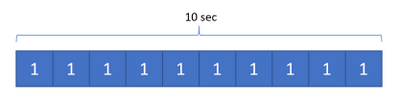Fig. A

Fig. B shows the same calculation split into 10 smaller tasks, so it only takes one second to run. Anaplan servers can use over 100 threads to improve efficiency like this.Fig. B

## Testing

In testing, I found that a CUMULATE over 2 million cells took around 0.5 seconds and a 20 million cell count took about 5.5 seconds. As the cell count goes up further, the duration increases. A 50 million cell count may take between 40 to 50 seconds. As the cell count grows the calculation and memory handling grows less efficient, so we don’t quite have a linear relationship between cell count and duration.

The advice, then, is that CUMULATE over lists is okay for a few million cells to keep the calculation less than a second. Bear in mind that the calculation is likely to be part of a calculation chain with other line items dependent on the value calculated in the cumulate—meaning this may add a wait of up to a second for those calculations, adding to the overall duration.

For anything larger, I recommend using CUMULATE over time instead. This function can be multi-threaded, and each period in the timescale is a separate calculation task in comparison to the single large tasks in the cumulate over list. This means it can be a lot more efficient, including lots of smaller calculations that can be split up versus one single calculation.

Here’s some data I ran for a test:

 Cell Count Duration (sec) % decrease CUMULATE over list 84,800,000 29.82 CUMULATE over time 83,200,000 1.01 96.6

## Analysis

If we look at the calculation analysis on this, we can see that the Cumulate over Time calculation actually does more work. The total calculation time is much higher. There is also the additional time needed for a lookup calculation (New Value) to take the calculated value from the time-based line item back to the original format.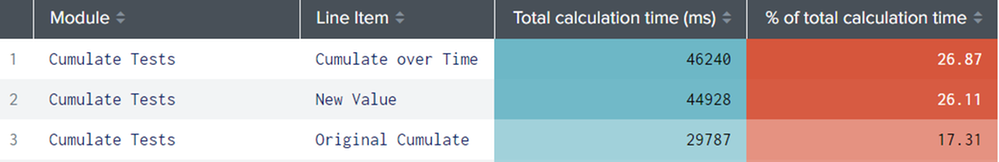When we take the multi-threading into account, the extra processing does not seem so bad. The duration here is seconds.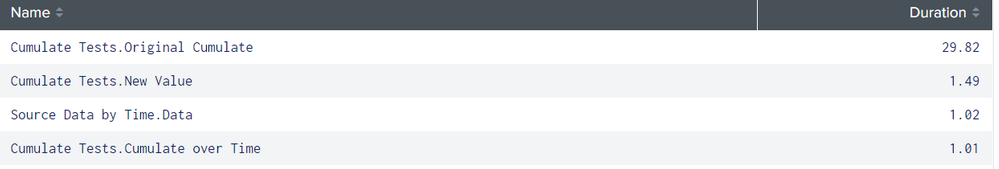The Source Data by Time line item here is used to take the original data into a time-based module via a mapping (so we can cumulate it). Even with the extra two calculations, the mapping lookups, the overall calculation time is much less than the single-threaded Original Cumulate line item (doing the cumulate over a list).

## Workaround

The reason for doing the cumulate over a list is because the source data is dimensioned by lists and not over time. In the model I used for this testing, one of the lists represented weeks, and fortunately, there were 52 list items. This allows us an easy mapping to actual weeks on a one-year time range.

The cumulate over list line item has this formula:

CUMULATE(Source Data.Values, FALSE, Custom Week)

It is cumulating the values from Source Data.Values over the Custom Week list.

The original source data is dimensioned by three lists: Custom Week, Order, Product.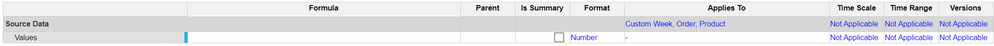The data needs to be put into a time-based module using a mapping via the list that is being used for the original cumulate (Custom Week).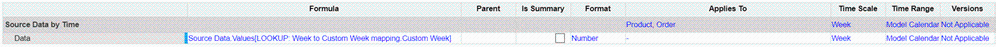We now have the data in this module dimensioned by three dimensions, replicating the original source module but with a time dimension replacing Custom Week.

The mapping module has a line item dimensioned by a 1yr time scale and week periods. The Custom Week values are mapped in list order to the timescale weeks. This could be any list, it just happened that the model I had was a list representing weeks.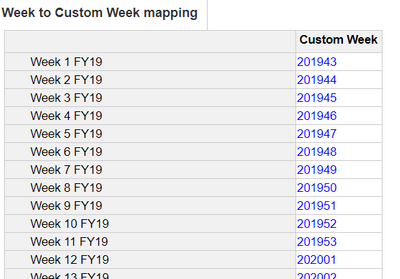This allows us to look up the source data matching the timescale week to the mapped Custom Week value:

Source Data.Values[LOOKUP: Week to Custom Week mapping.Custom Week]

A new line item can be created that is dimensioned the same as this new source data so that it can be cumulated over time:

CUMULATE(Source Data by Time.Data)

To put the data back into the same dimensions as the original line item, we need to do another lookup with a reverse mapping:

Cumulate over Time[LOOKUP: Custom Week to Week mapping.Week]

The mapping module is the opposite of the previous mapping, a line item dimensioned by Custom Week with the values matching the previous mappings.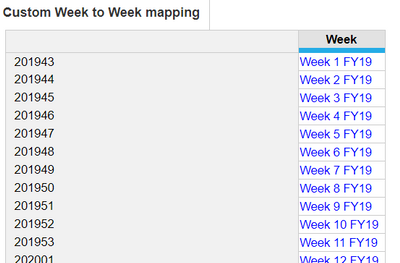Here are the three line items in blueprint view: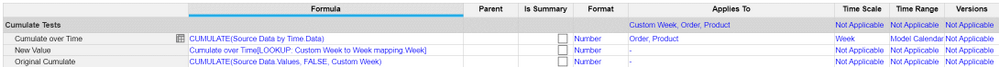As a straightforward workaround, you just need to map the list you intended to cumulate over to time periods and then cumulate over time (with a mapping lookup if needed to put the value in the desired dimensionality). The type of period doesn’t matter. It’s just a mapping to replicate the list order using time.

Tagged: